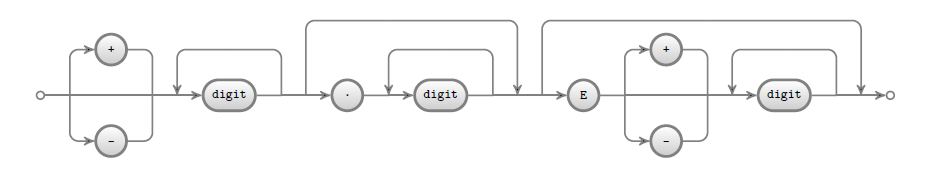# grammar2diagram(mos's homepage)

This project provides a tool to automatically generate a TikZ (LaTeX) diagram from a EBNF grammar.

The `EBNF` grammar:
```("+" | "" | "-") ( "digit" )+ ( "." ( "digit" )+ )? ( "E" ("+" | "" | "-") ( "digit" )+ )? ```
When processed by `grammar2diagram` generates the following `LaTeX` diagram: